waypointTrajectory

Waypoint trajectory generator

Description

The waypointTrajectory System object™ generates trajectories using specified waypoints. When you create the System object, you can optionally specify the time of arrival, velocity, and orientation at each waypoint. See Algorithms for more details.

To generate a trajectory from waypoints:

1. Create the waypointTrajectory object and set its properties.

2. Call the object as if it were a function.

Creation

Description

example

trajectory = waypointTrajectory returns a System object, trajectory, that generates a trajectory based on default stationary waypoints.

example

trajectory = waypointTrajectory(Waypoints,TimeOfArrival) specifies the Waypoints that the generated trajectory passes through and the TimeOfArrival at each waypoint.

example

trajectory = waypointTrajectory(Waypoints,TimeOfArrival,Name,Value) sets each creation argument or property Name to the specified Value. Unspecified properties and creation arguments have default or inferred values.

Example: trajectory = waypointTrajectory([10,10,0;20,20,0;20,20,10],[0,0.5,10]) creates a waypoint trajectory System object, trajectory, that starts at waypoint [10,10,0], and then passes through [20,20,0] after 0.5 seconds and [20,20,10] after 10 seconds.

Creation Arguments

Creation arguments are properties which are set during creation of the System object and cannot be modified later. If you do not explicitly set a creation argument value, the property value is inferred.

If you specify any creation argument, then you must specify both the Waypoints and TimeOfArrival creation arguments. You can specify Waypoints and TimeOfArrival as value-only arguments or name-value pairs.

Properties

expand all

Unless otherwise indicated, properties are nontunable, which means you cannot change their values after calling the object. Objects lock when you call them, and the release function unlocks them.

If a property is tunable, you can change its value at any time.

Sample rate of trajectory in Hz, specified as a positive scalar.

Tunable: Yes

Data Types: double

Number of samples per output frame, specified as a positive scalar integer.

Tunable: Yes

Data Types: double

Positions in the navigation coordinate system in meters, specified as an N-by-3 matrix. The columns of the matrix correspond to the first, second, and third axes, respectively. The rows of the matrix, N, correspond to individual waypoints.

Dependencies

To set this property, you must also set valid values for the TimeOfArrival property.

Data Types: double

Time corresponding to arrival at each waypoint in seconds, specified as an N-element column vector. The first element of TimeOfArrival must be 0. The number of samples, N, must be the same as the number of samples (rows) defined by Waypoints.

Dependencies

To set this property, you must also set valid values for the Waypoints property.

Data Types: double

Velocity in the navigation coordinate system at each way point in meters per second, specified as an N-by-3 matrix. The columns of the matrix correspond to the first, second, and third axes, respectively. The number of samples, N, must be the same as the number of samples (rows) defined by Waypoints.

If the velocity is specified as a non-zero value, the object automatically calculates the course of the trajectory. If the velocity is specified as zero, the object infers the course of the trajectory from adjacent waypoints.

Dependencies

To set this property, you must also set valid values for the Waypoints and TimeOfArrival properties.

Data Types: double

Horizontal direction of travel, specified as an N-element real vector in degrees. The number of samples, N, must be the same as the number of samples (rows) defined by Waypoints. If neither Velocities nor Course is specified, course is inferred from the waypoints.

Dependencies

To set this property, the Velocities property must not be specified in object creation.

Data Types: double

Groundspeed at each waypoint, specified as an N-element real vector in m/s. If the property is not specified, it is inferred from the waypoints. The number of samples, N, must be the same as the number of samples (rows) defined by Waypoints.

Dependencies

To set this property, the Velocities property must not be specified at object creation.

Data Types: double

Climb Rate at each waypoint, specified as an N-element real vector in degrees. The number of samples, N, must be the same as the number of samples (rows) defined by Waypoints. If neither Velocities nor Course is specified, climbrate is inferred from the waypoints.

Dependencies

To set this property, the Velocities property must not be specified at object creation.

Data Types: double

Orientation at each waypoint, specified as an N-element quaternion column vector or 3-by-3-by-N array of real numbers. The number of quaternions or rotation matrices, N, must be the same as the number of samples (rows) defined by Waypoints.

If Orientation is specified by quaternions, the underlying class must be double.

Dependencies

To set this property, you must also set valid values for the Waypoints and TimeOfArrival properties.

Data Types: quaternion | double

Align pitch angle with the direction of motion, specified as true or false. When specified as true, the pitch angle automatically aligns with the direction of motion. If specified as false, the pitch angle is set to zero (level orientation).

Dependencies

To set this property, the Orientation property must not be specified at object creation.

Align roll angle to counteract the centripetal force, specified as true or false. When specified as true, the roll angle automatically counteract the centripetal force. If specified as false, the roll angle is set to zero (flat orientation).

Dependencies

To set this property, the Orientation property must not be specified at object creation.

Reference frame of the trajectory, specified as 'NED' (North-East-Down) or 'ENU' (East-North-Up).

Usage

Description

example

[position,orientation,velocity,acceleration,angularVelocity] = trajectory() outputs a frame of trajectory data based on specified creation arguments and properties.

Output Arguments

expand all

Position in the local navigation coordinate system in meters, returned as an M-by-3 matrix.

M is specified by the SamplesPerFrame property.

Data Types: double

Orientation in the local navigation coordinate system, returned as an M-by-1 quaternion column vector or a 3-by-3-by-M real array.

Each quaternion or 3-by-3 rotation matrix is a frame rotation from the local navigation coordinate system to the current body coordinate system.

M is specified by the SamplesPerFrame property.

Data Types: double

Velocity in the local navigation coordinate system in meters per second, returned as an M-by-3 matrix.

M is specified by the SamplesPerFrame property.

Data Types: double

Acceleration in the local navigation coordinate system in meters per second squared, returned as an M-by-3 matrix.

M is specified by the SamplesPerFrame property.

Data Types: double

Angular velocity in the local navigation coordinate system in radians per second, returned as an M-by-3 matrix.

M is specified by the SamplesPerFrame property.

Data Types: double

Object Functions

To use an object function, specify the System object as the first input argument. For example, to release system resources of a System object named obj, use this syntax:

release(obj)

expand all

 waypointInfo Get waypoint information table lookupPose Obtain pose information for certain time perturbations Perturbation defined on object perturb Apply perturbations to object
 clone Create duplicate System object step Run System object algorithm release Release resources and allow changes to System object property values and input characteristics reset Reset internal states of System object isDone End-of-data status

Examples

collapse all

trajectory = waypointTrajectory
trajectory =
waypointTrajectory with properties:

SampleRate: 100
SamplesPerFrame: 1
Waypoints: [2x3 double]
TimeOfArrival: [2x1 double]
Velocities: [2x3 double]
Course: [2x1 double]
GroundSpeed: [2x1 double]
ClimbRate: [2x1 double]
Orientation: [2x1 quaternion]
AutoPitch: 0
AutoBank: 0
ReferenceFrame: 'NED'

Inspect the default waypoints and times of arrival by calling waypointInfo. By default, the waypoints indicate a stationary position for one second.

waypointInfo(trajectory)
ans=2×2 table
TimeOfArrival     Waypoints
_____________    ___________

0          0    0    0
1          0    0    0

Create a square trajectory and examine the relationship between waypoint constraints, sample rate, and the generated trajectory.

Create a square trajectory by defining the vertices of the square. Define the orientation at each waypoint as pointing in the direction of motion. Specify a 1 Hz sample rate and use the default SamplesPerFrame of 1.

waypoints = [0,0,0; ... % Initial position
0,1,0; ...
1,1,0; ...
1,0,0; ...
0,0,0];    % Final position

toa = 0:4; % time of arrival

orientation = quaternion([0,0,0; ...
45,0,0; ...
135,0,0; ...
225,0,0; ...
0,0,0], ...
'eulerd','ZYX','frame');

trajectory = waypointTrajectory(waypoints, ...
'TimeOfArrival',toa, ...
'Orientation',orientation, ...
'SampleRate',1);

Create a figure and plot the initial position of the platform.

figure(1)
plot(waypoints(1,1),waypoints(1,2),'b*')
title('Position')
axis([-1,2,-1,2])
axis square
xlabel('X')
ylabel('Y')
grid on
hold onIn a loop, step through the trajectory to output the current position and current orientation. Plot the current position and log the orientation. Use pause to mimic real-time processing.

orientationLog = zeros(toa(end)*trajectory.SampleRate,1,'quaternion');
count = 1;
while ~isDone(trajectory)
[currentPosition,orientationLog(count)] = trajectory();

plot(currentPosition(1),currentPosition(2),'bo')

pause(trajectory.SamplesPerFrame/trajectory.SampleRate)
count = count + 1;
end
hold off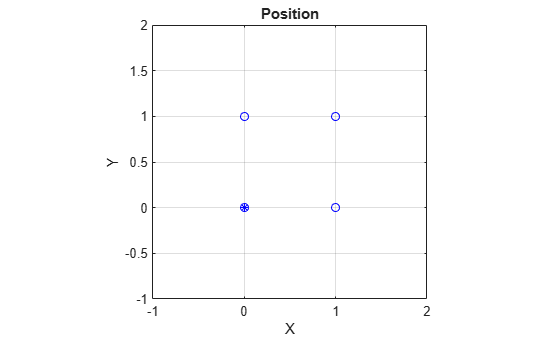Convert the orientation quaternions to Euler angles for easy interpretation, and then plot orientation over time.

figure(2)
eulerAngles = eulerd([orientation(1);orientationLog],'ZYX','frame');
plot(toa,eulerAngles(:,1),'ko', ...
toa,eulerAngles(:,2),'bd', ...
toa,eulerAngles(:,3),'r.');
title('Orientation Over Time')
legend('Rotation around Z-axis','Rotation around Y-axis','Rotation around X-axis')
xlabel('Time (seconds)')
ylabel('Rotation (degrees)')
grid on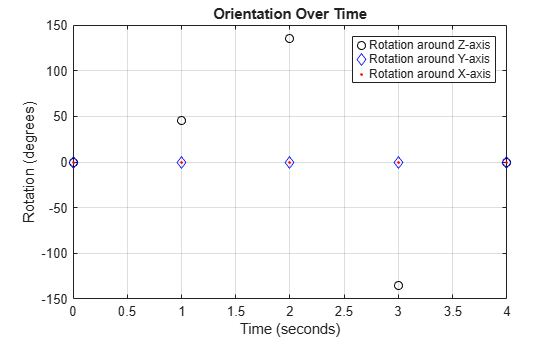So far, the trajectory object has only output the waypoints that were specified during construction. To interpolate between waypoints, increase the sample rate to a rate faster than the time of arrivals of the waypoints. Set the trajectory sample rate to 100 Hz and call reset.

trajectory.SampleRate = 100;
reset(trajectory)

Create a figure and plot the initial position of the platform. In a loop, step through the trajectory to output the current position and current orientation. Plot the current position and log the orientation. Use pause to mimic real-time processing.

figure(1)
plot(waypoints(1,1),waypoints(1,2),'b*')
title('Position')
axis([-1,2,-1,2])
axis square
xlabel('X')
ylabel('Y')
grid on
hold on

orientationLog = zeros(toa(end)*trajectory.SampleRate,1,'quaternion');
count = 1;
while ~isDone(trajectory)
[currentPosition,orientationLog(count)] = trajectory();

plot(currentPosition(1),currentPosition(2),'bo')

pause(trajectory.SamplesPerFrame/trajectory.SampleRate)
count = count + 1;
end
hold off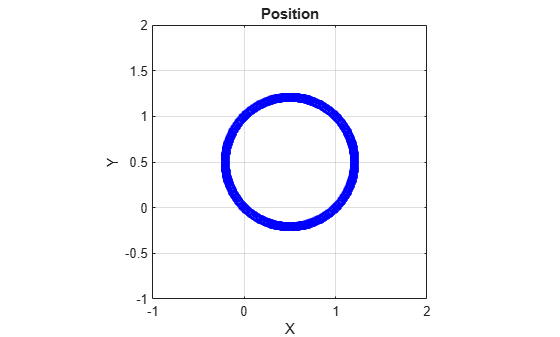The trajectory output now appears circular. This is because the waypointTrajectory System object™ minimizes the acceleration and angular velocity when interpolating, which results in smoother, more realistic motions in most scenarios.

Convert the orientation quaternions to Euler angles for easy interpretation, and then plot orientation over time. The orientation is also interpolated.

figure(2)
eulerAngles = eulerd([orientation(1);orientationLog],'ZYX','frame');
t = 0:1/trajectory.SampleRate:4;
plot(t,eulerAngles(:,1),'ko', ...
t,eulerAngles(:,2),'bd', ...
t,eulerAngles(:,3),'r.');
title('Orientation Over Time')
legend('Rotation around Z-axis','Rotation around Y-axis','Rotation around X-axis')
xlabel('Time (seconds)')
ylabel('Rotation (degrees)')
grid onThe waypointTrajectory algorithm interpolates the waypoints to create a smooth trajectory. To return to the square trajectory, provide more waypoints, especially around sharp changes. To track corresponding times, waypoints, and orientation, specify all the trajectory info in a single matrix.

% Time, Waypoint, Orientation
trajectoryInfo = [0,   0,0,0,    0,0,0; ... % Initial position
0.1, 0,0.1,0,  0,0,0; ...

0.9, 0,0.9,0,  0,0,0; ...
1,   0,1,0,    45,0,0; ...
1.1, 0.1,1,0,  90,0,0; ...

1.9, 0.9,1,0,  90,0,0; ...
2,   1,1,0,    135,0,0; ...
2.1, 1,0.9,0,  180,0,0; ...

2.9, 1,0.1,0,  180,0,0; ...
3,   1,0,0,    225,0,0; ...
3.1, 0.9,0,0,  270,0,0; ...

3.9, 0.1,0,0,  270,0,0; ...
4,   0,0,0,    270,0,0];    % Final position

trajectory = waypointTrajectory(trajectoryInfo(:,2:4), ...
'TimeOfArrival',trajectoryInfo(:,1), ...
'Orientation',quaternion(trajectoryInfo(:,5:end),'eulerd','ZYX','frame'), ...
'SampleRate',100);

Create a figure and plot the initial position of the platform. In a loop, step through the trajectory to output the current position and current orientation. Plot the current position and log the orientation. Use pause to mimic real-time processing.

figure(1)
plot(waypoints(1,1),waypoints(1,2),'b*')
title('Position')
axis([-1,2,-1,2])
axis square
xlabel('X')
ylabel('Y')
grid on
hold on

orientationLog = zeros(toa(end)*trajectory.SampleRate,1,'quaternion');
count = 1;
while ~isDone(trajectory)
[currentPosition,orientationLog(count)] = trajectory();

plot(currentPosition(1),currentPosition(2),'bo')

pause(trajectory.SamplesPerFrame/trajectory.SampleRate)
count = count+1;
end
hold offThe trajectory output now appears more square-like, especially around the vertices with waypoints.

Convert the orientation quaternions to Euler angles for easy interpretation, and then plot orientation over time.

figure(2)
eulerAngles = eulerd([orientation(1);orientationLog],'ZYX','frame');
t = 0:1/trajectory.SampleRate:4;
eulerAngles = plot(t,eulerAngles(:,1),'ko', ...
t,eulerAngles(:,2),'bd', ...
t,eulerAngles(:,3),'r.');
title('Orientation Over Time')
legend('Rotation around Z-axis', ...
'Rotation around Y-axis', ...
'Rotation around X-axis', ...
'Location', 'SouthWest')
xlabel('Time (seconds)')
ylabel('Rotation (degrees)')
grid on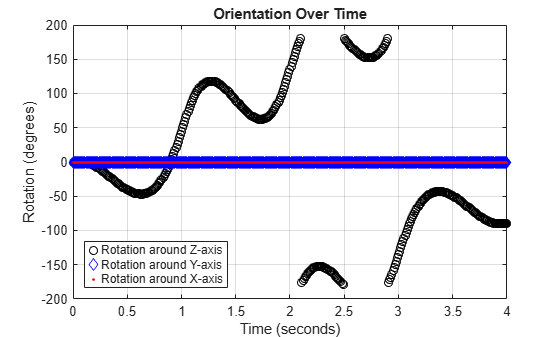This example shows how to create an arc trajectory using the waypointTrajectory System object™. waypointTrajectory creates a path through specified waypoints that minimizes acceleration and angular velocity. After creating an arc trajectory, you restrict the trajectory to be within preset bounds.

Create an Arc Trajectory

Define a constraints matrix consisting of waypoints, times of arrival, and orientation for an arc trajectory. The generated trajectory passes through the waypoints at the specified times with the specified orientation. The waypointTrajectory System object requires orientation to be specified using quaternions or rotation matrices. Convert the Euler angles saved in the constrains matrix to quaternions when specifying the Orientation property.

% Arrival, Waypoints, Orientation
constraints = [0,    20,20,0,    90,0,0;
3,    50,20,0,    90,0,0;
4,    58,15.5,0,  162,0,0;
5.5,  59.5,0,0    180,0,0];

trajectory = waypointTrajectory(constraints(:,2:4), ...
'TimeOfArrival',constraints(:,1), ...
'Orientation',quaternion(constraints(:,5:7),'eulerd','ZYX','frame'));

Call waypointInfo on trajectory to return a table of your specified constraints. The creation properties Waypoints, TimeOfArrival, and Orientation are variables of the table. The table is convenient for indexing while plotting.

tInfo = waypointInfo(trajectory)
tInfo =

4x3 table

TimeOfArrival         Waypoints            Orientation
_____________    ____________________    ________________

0           20      20       0    {1x1 quaternion}
3           50      20       0    {1x1 quaternion}
4           58    15.5       0    {1x1 quaternion}
5.5         59.5       0       0    {1x1 quaternion}

The trajectory object outputs the current position, velocity, acceleration, and angular velocity at each call. Call trajectory in a loop and plot the position over time. Cache the other outputs.

figure(1)
plot(tInfo.Waypoints(1,1),tInfo.Waypoints(1,2),'b*')
title('Position')
axis([20,65,0,25])
xlabel('North')
ylabel('East')
grid on
daspect([1 1 1])
hold on

orient = zeros(tInfo.TimeOfArrival(end)*trajectory.SampleRate,1,'quaternion');
vel = zeros(tInfo.TimeOfArrival(end)*trajectory.SampleRate,3);
acc = vel;
angVel = vel;

count = 1;
while ~isDone(trajectory)
[pos,orient(count),vel(count,:),acc(count,:),angVel(count,:)] = trajectory();

plot(pos(1),pos(2),'bo')

pause(trajectory.SamplesPerFrame/trajectory.SampleRate)
count = count + 1;
end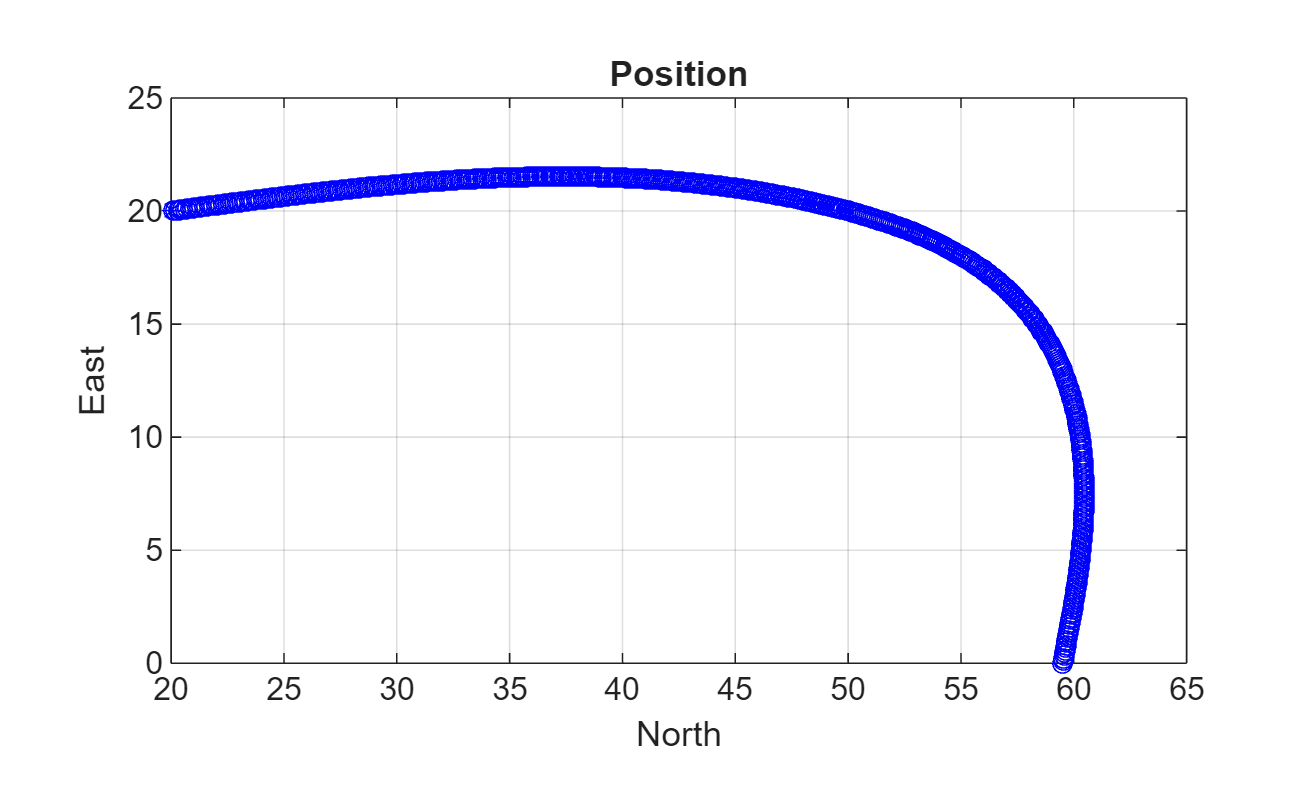Inspect the orientation, velocity, acceleration, and angular velocity over time. The waypointTrajectory System object™ creates a path through the specified constraints that minimized acceleration and angular velocity.

figure(2)
timeVector = 0:(1/trajectory.SampleRate):tInfo.TimeOfArrival(end);
eulerAngles = eulerd([tInfo.Orientation{1};orient],'ZYX','frame');
plot(timeVector,eulerAngles(:,1), ...
timeVector,eulerAngles(:,2), ...
timeVector,eulerAngles(:,3));
title('Orientation Over Time')
legend('Rotation around Z-axis', ...
'Rotation around Y-axis', ...
'Rotation around X-axis', ...
'Location','southwest')
xlabel('Time (seconds)')
ylabel('Rotation (degrees)')
grid on

figure(3)
plot(timeVector(2:end),vel(:,1), ...
timeVector(2:end),vel(:,2), ...
timeVector(2:end),vel(:,3));
title('Velocity Over Time')
legend('North','East','Down')
xlabel('Time (seconds)')
ylabel('Velocity (m/s)')
grid on

figure(4)
plot(timeVector(2:end),acc(:,1), ...
timeVector(2:end),acc(:,2), ...
timeVector(2:end),acc(:,3));
title('Acceleration Over Time')
legend('North','East','Down','Location','southwest')
xlabel('Time (seconds)')
ylabel('Acceleration (m/s^2)')
grid on

figure(5)
plot(timeVector(2:end),angVel(:,1), ...
timeVector(2:end),angVel(:,2), ...
timeVector(2:end),angVel(:,3));
title('Angular Velocity Over Time')
legend('North','East','Down')
xlabel('Time (seconds)')
grid on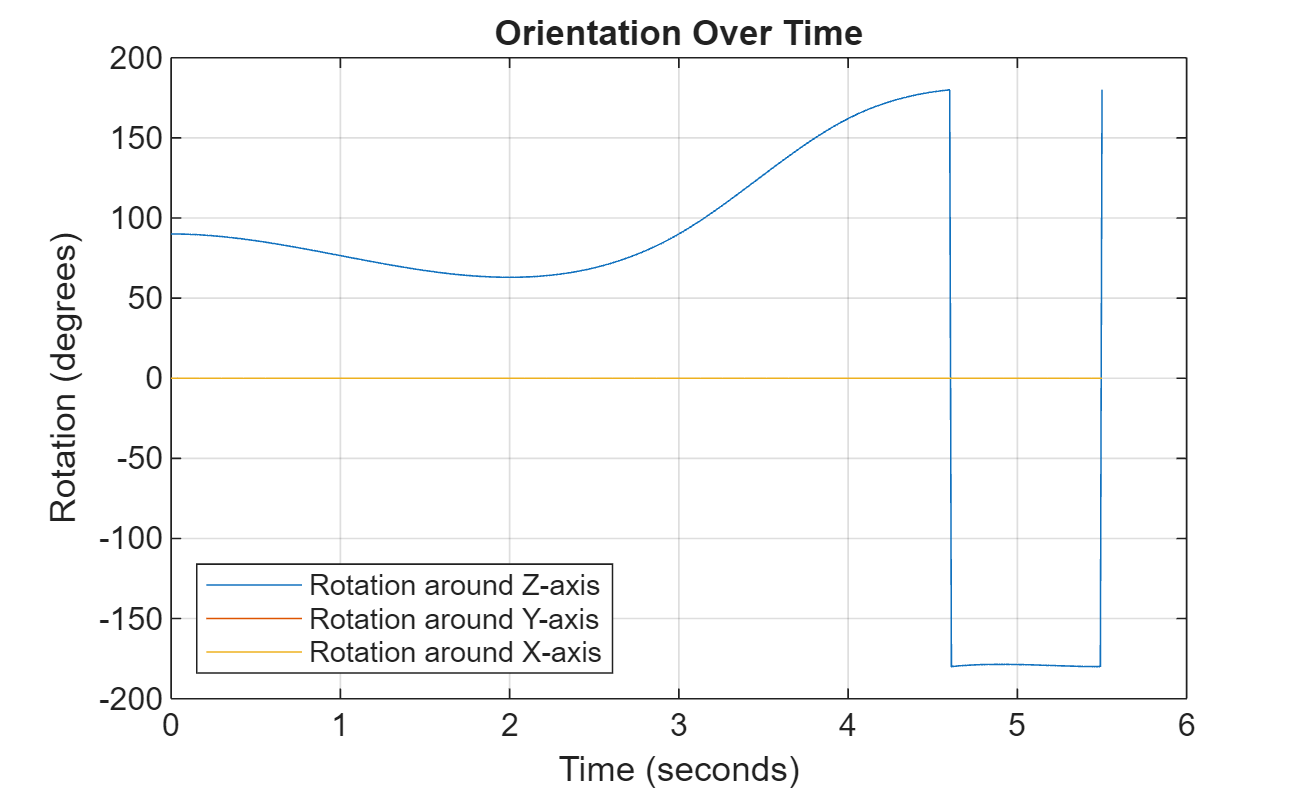Restrict Arc Trajectory Within Preset Bounds

You can specify additional waypoints to create trajectories within given bounds. Create upper and lower bounds for the arc trajectory.

figure(1)
xUpperBound = [(20:50)';50+10*sin(0:0.1:pi/2)';60*ones(11,1)];
yUpperBound = [20.5.*ones(31,1);10.5+10*cos(0:0.1:pi/2)';(10:-1:0)'];

xLowerBound = [(20:49)';50+9*sin(0:0.1:pi/2)';59*ones(11,1)];
yLowerBound = [19.5.*ones(30,1);10.5+9*cos(0:0.1:pi/2)';(10:-1:0)'];

plot(xUpperBound,yUpperBound,'r','LineWidth',2);
plot(xLowerBound,yLowerBound,'r','LineWidth',2)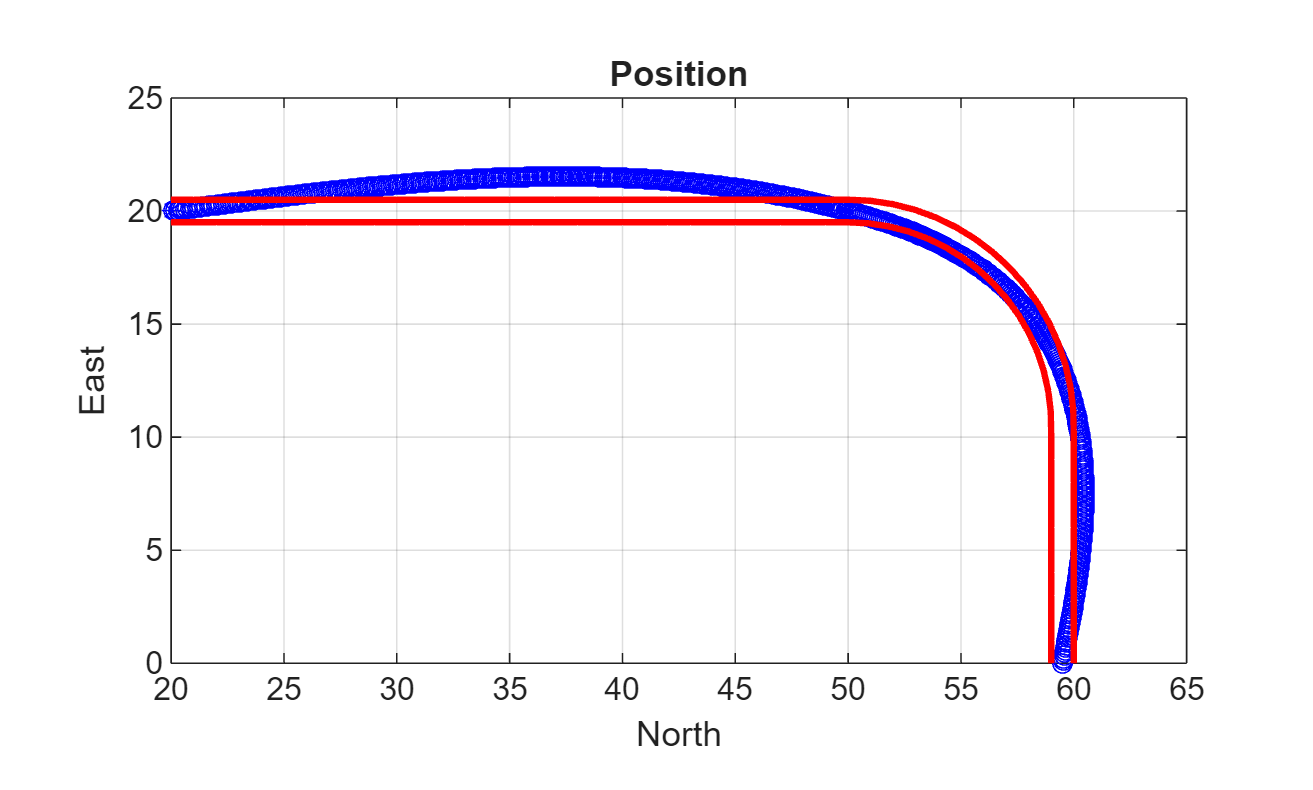To create a trajectory within the bounds, add additional waypoints. Create a new waypointTrajectory System object™, and then call it in a loop to plot the generated trajectory. Cache the orientation, velocity, acceleration, and angular velocity output from the trajectory object.

% Time,  Waypoint,     Orientation
constraints = [0,    20,20,0,      90,0,0;
1.5,  35,20,0,      90,0,0;
2.5   45,20,0,      90,0,0;
3,    50,20,0,      90,0,0;
3.3,  53,19.5,0,    108,0,0;
3.6,  55.5,18.25,0, 126,0,0;
3.9,  57.5,16,0,    144,0,0;
4.2,  59,14,0,      162,0,0;
4.5,  59.5,10,0     180,0,0;
5,    59.5,5,0      180,0,0;
5.5,  59.5,0,0      180,0,0];

trajectory = waypointTrajectory(constraints(:,2:4), ...
'TimeOfArrival',constraints(:,1), ...
'Orientation',quaternion(constraints(:,5:7),'eulerd','ZYX','frame'));
tInfo = waypointInfo(trajectory);

figure(1)
plot(tInfo.Waypoints(1,1),tInfo.Waypoints(1,2),'b*')

count = 1;
while ~isDone(trajectory)
[pos,orient(count),vel(count,:),acc(count,:),angVel(count,:)] = trajectory();

plot(pos(1),pos(2),'gd')

pause(trajectory.SamplesPerFrame/trajectory.SampleRate)
count = count + 1;
end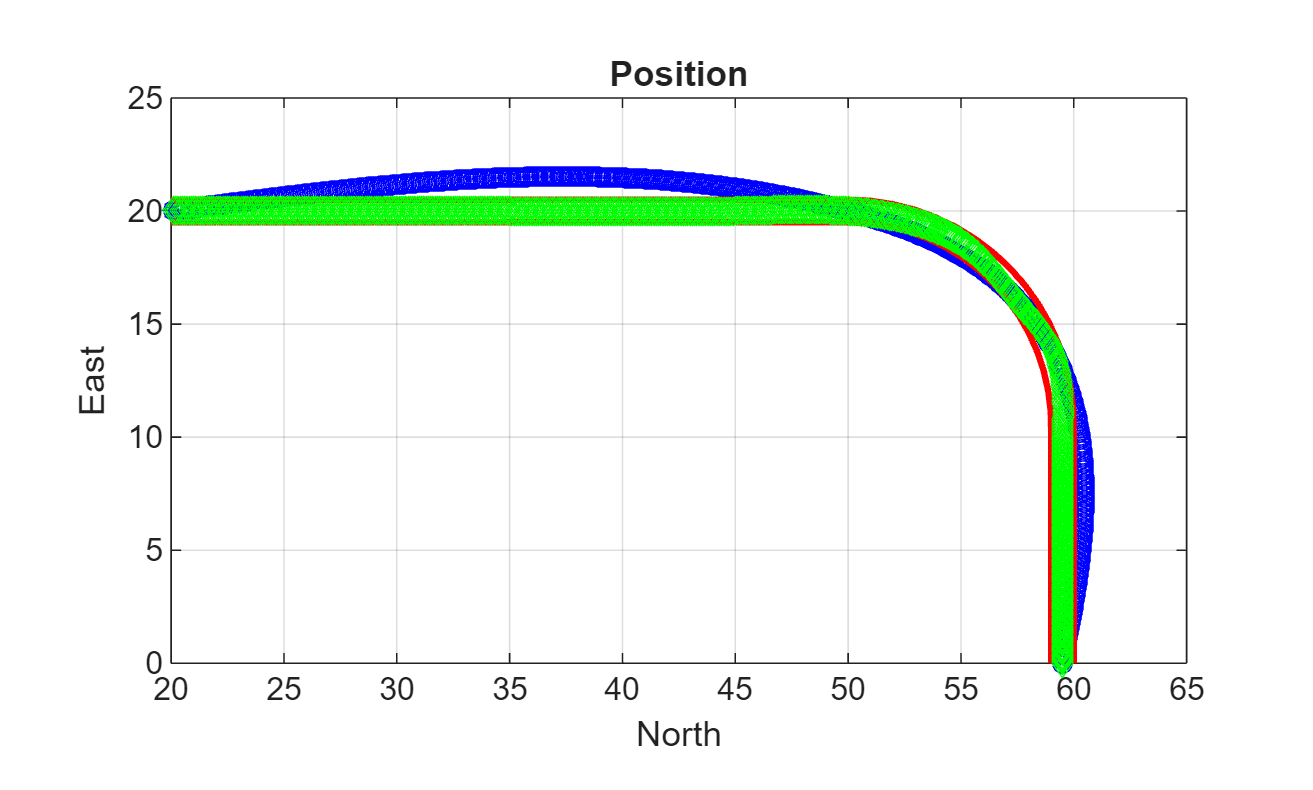The generated trajectory now fits within the specified boundaries. Visualize the orientation, velocity, acceleration, and angular velocity of the generated trajectory.

figure(2)
timeVector = 0:(1/trajectory.SampleRate):tInfo.TimeOfArrival(end);
eulerAngles = eulerd(orient,'ZYX','frame');
plot(timeVector(2:end),eulerAngles(:,1), ...
timeVector(2:end),eulerAngles(:,2), ...
timeVector(2:end),eulerAngles(:,3));
title('Orientation Over Time')
legend('Rotation around Z-axis', ...
'Rotation around Y-axis', ...
'Rotation around X-axis', ...
'Location','southwest')
xlabel('Time (seconds)')
ylabel('Rotation (degrees)')
grid on

figure(3)
plot(timeVector(2:end),vel(:,1), ...
timeVector(2:end),vel(:,2), ...
timeVector(2:end),vel(:,3));
title('Velocity Over Time')
legend('North','East','Down')
xlabel('Time (seconds)')
ylabel('Velocity (m/s)')
grid on

figure(4)
plot(timeVector(2:end),acc(:,1), ...
timeVector(2:end),acc(:,2), ...
timeVector(2:end),acc(:,3));
title('Acceleration Over Time')
legend('North','East','Down')
xlabel('Time (seconds)')
ylabel('Acceleration (m/s^2)')
grid on

figure(5)
plot(timeVector(2:end),angVel(:,1), ...
timeVector(2:end),angVel(:,2), ...
timeVector(2:end),angVel(:,3));
title('Angular Velocity Over Time')
legend('North','East','Down')
xlabel('Time (seconds)')
grid on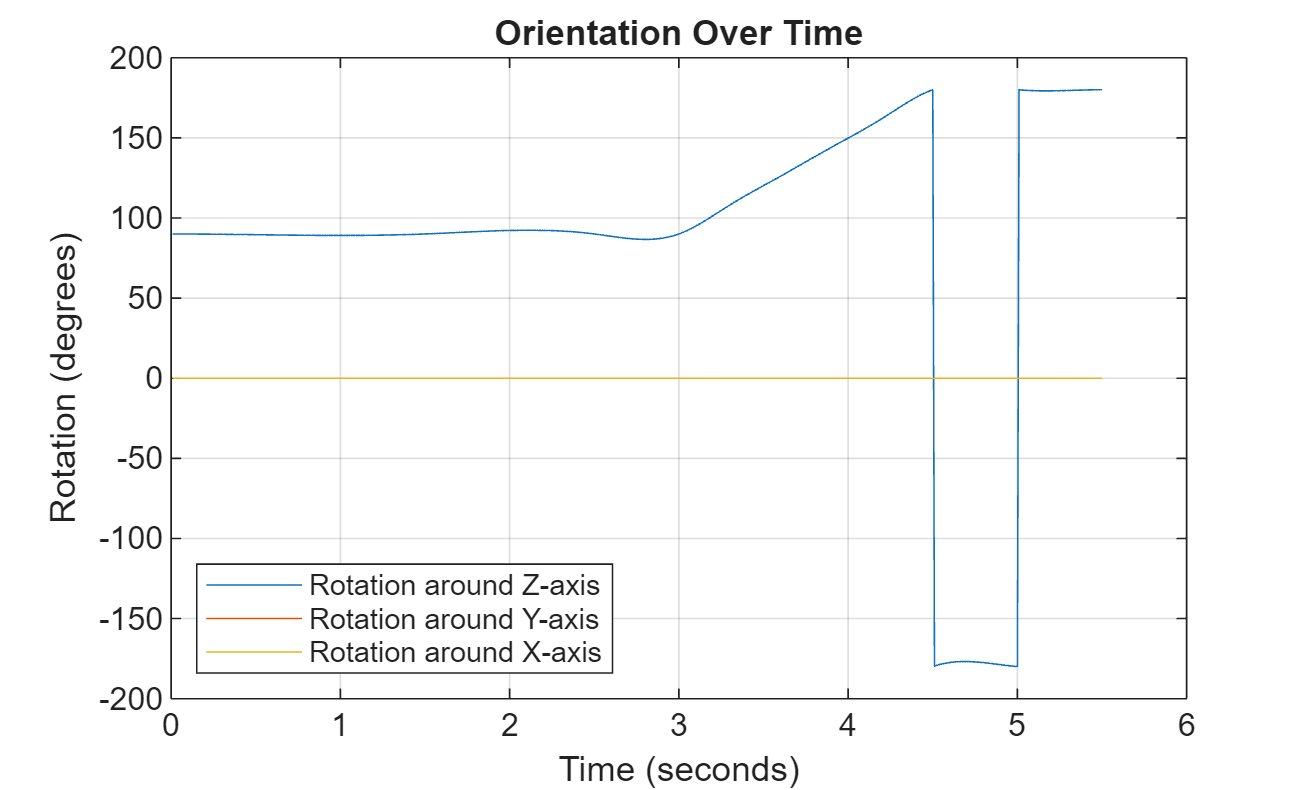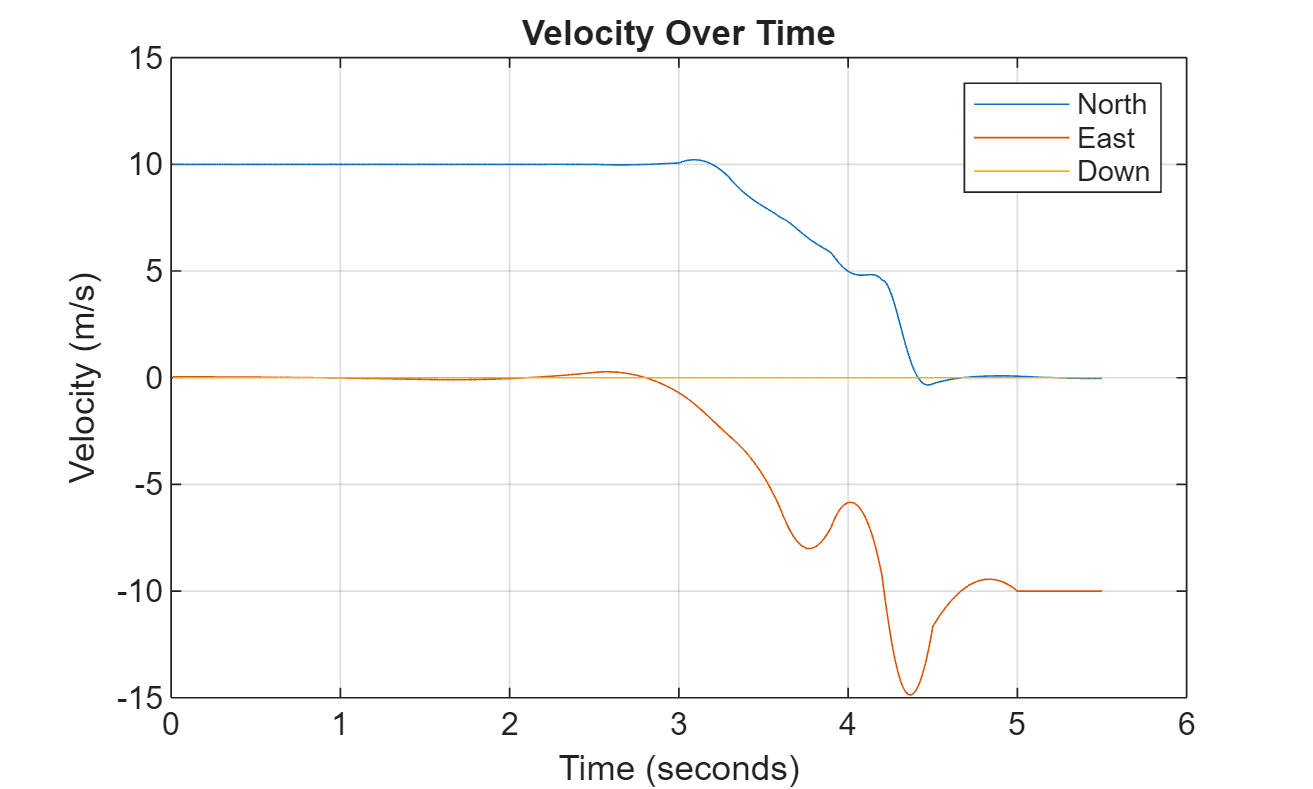Note that while the generated trajectory now fits within the spatial boundaries, the acceleration and angular velocity of the trajectory are somewhat erratic. This is due to over-specifying waypoints.

Algorithms

The waypointTrajectory System object defines a trajectory that smoothly passes through waypoints. The trajectory connects the waypoints through an interpolation that assumes the gravity direction expressed in the trajectory reference frame is constant. Generally, you can use waypointTrajectory to model platform or vehicle trajectories within a hundreds of kilometers distance span.

The planar path of the trajectory (the x-y plane projection) consists of piecewise, clothoid curves. The curvature of the curve between two consecutive waypoints varies linearly with the curve length between them. The tangent direction of the path at each waypoint is chosen to minimize discontinuities in the curvature, unless the course is specified explicitly via the Course property or implicitly via the Velocities property. Once the path is established, the object uses cubic Hermite interpolation to compute the location of the vehicle throughout the path as a function of time and the planar distance travelled.

The normal component (z-component) of the trajectory is subsequently chosen to satisfy a shape-preserving piecewise spline (PCHIP) unless the climb rate is specified explicitly via the ClimbRate property or the third column of the Velocities property. Choose the sign of the climb rate based on the selected ReferenceFrame:

• When an 'ENU' reference frame is selected, specifying a positive climb rate results in an increasing value of z.

• When an 'NED' reference frame is selected, specifying a positive climb rate results in a decreasing value of z.

You can define the orientation of the vehicle through the path in two primary ways:

• If the Orientation property is specified, then the object uses a piecewise-cubic, quaternion spline to compute the orientation along the path as a function of time.

• If the Orientation property is not specified, then the yaw of the vehicle is always aligned with the path. The roll and pitch are then governed by the AutoBank and AutoPitch property values, respectively.

AutoBankAutoPitchDescription
falsefalseThe vehicle is always level (zero pitch and roll). This is typically used for large marine vessels.
falsetrueThe vehicle pitch is aligned with the path, and its roll is always zero. This is typically used for ground vehicles.
truefalseThe vehicle pitch and roll are chosen so that its local z-axis is aligned with the net acceleration (including gravity). This is typically used for rotary-wing craft.
truetrueThe vehicle roll is chosen so that its local transverse plane aligns with the net acceleration (including gravity). The vehicle pitch is aligned with the path. This is typically used for two-wheeled vehicles and fixed-wing aircraft.